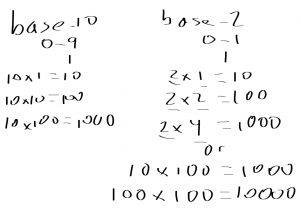# Homeschool Technology Lesson 3 – Binary (practice)

Today was more practice for homeschool technology lesson. We did more binary and discussed how to calculate what the value when adding a new column.

See: Previous homeschool posts

## Homeschool Technology Lesson 3 – Binary (practice)

So for base-10 (decimal), the first column values are 0-9. You know this because the “10” in base-10 tells you you can’t do ten with one column. So for ten, you need to add a new column: 10.Then each column is ten times the previous column value with a 1 in it.

10 x 1 = 10

10 x 10 = 100

so on

Then for binary, base-2, each column can go up to 1 and you multiple the previous column by 2 to get the next column.

0 = zero

1 = one

2 x 1 = 10 (two)

2 x 2 = 100 (four)

2 x 4 = 1000 (eight)

See the image for another way to write that – in binary.

In the image, see the underlined items. Those are ones I left blank and had them tell me what to fill in. I’d ask for the number but also make sure they knew where it came from.

So for decimal, I made sure they knew the ten came from “base-10.” Then the second value came from the value of the previous column.

I did this, erased it and did it again.

Math is a foreign language and it’s best learned through emersion and practice.

Same for binary, the two comes from “base-2” and the second number comes from the previous column’s value.

So just like with decimal adding a zero on the end makes the value go up by 10. In binary, adding a zero doubles the value.

Or the other way around, in decimal, multiplying by ten adds a zero. In binary, multiply by 2 adds a zero.

We’ll probably practice this a couple more times and move on to base-16 (hexadecimal). We’ll see – I’m not really planning this out. #wingingit